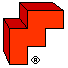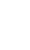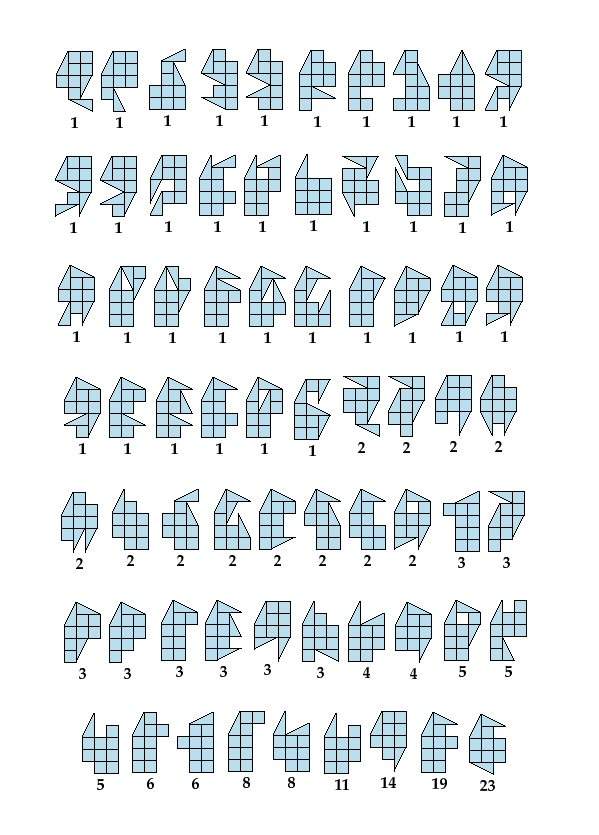Shardinaires-9:more challengesTwins Magic SquaresThis puzzle set of 9 tiles started out with a modest challenge: form them into each of the 12 pentominoes and each of the 5 tetrominoes. Simply put: make 5 squares = 4 squares. Then more questions arose: can they form convex polygons, and how many? How about symmetrical shapes? There are hundreds. How about models of each tile? Found all — their full list is in the booklet. How about subset symmetries? Hundreds of those turned up. How about "twins", splitting the 9 tiles into two identical shapes? So many of those turned up that they didn't all fit into the booklet, so we share them here.

Twins

The tables below are a complete catalog of all twins that fit into 3x5, 4x4, 2x6, and 2x7 outlines. The number next to each figure is how many solutions that shape can have. Build two identical copies of each, simultaneously, using all 9 tiles. You'll never run out of challenges.
 The 163 twins of size 4x4 that have only 1 solution:The 102 twins of size 4x4 that have 2 to 19 solutions:The 100 twins of size 3x5 that have 1 to 24 solutions:The 69 twins of size 5x3 with 1 to 23 solutions:The 30 twins of sizes 2x6, 2x7 and 3x4, with 1 to 18 solutions:Magic Squares Arrange the 9 Shardinaires-9 tiles in a 3x3 array so that every straight row of three—horizontal or vertical—can form a symmetrical figure. There are a total of 29 ways to build a magic 3x3. Twelve of them are in the booklet. Here are the other 17. That's a total of 174 symmetrical trios.Amazing, aren't they? Pick any square and find all 6 of its solutions. Yes, a human can do it without a computer. Surprise yourself.